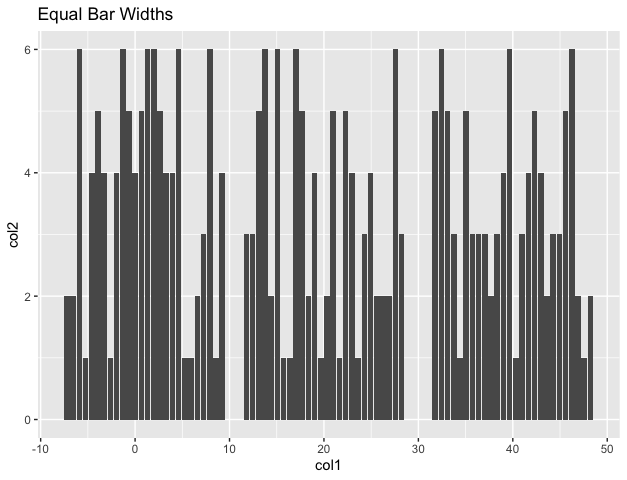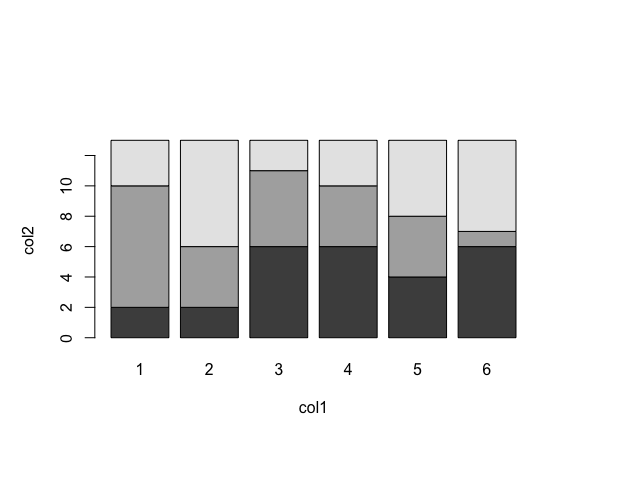# How to Make Grouped Bar Plot with Same Bar Width in R

• Last Updated : 17 Oct, 2021

In this article, we will discuss How to Make Grouped Bar Plot with the Same Bar Width in R Programming Language.

## Method  1 : Using position_dodge2(preserve = “single”)

The geom_col() method can be used to add positions to the graph. Dodging preserves the vertical position of an geom while adjusting the horizontal position. The position_dodge2 method is used to work with bars and rectangle.

Syntax :

`position_dodge2(width = NULL, preserve = c("total", "single"))`

Parameters:

• width – Dodging width, when different to the width of the individual elements.
• preserve – Indicator of whether of not dodging should preserve the total width of all elements at a position or a single element.

Example:

## Python3

 `library(``"ggplot2"``) ` `library(``"ggforce"``) ` ` `  ` `  `# creating a data frame ` `df < ``-` `data.frame(col1``=``sample(rep(c(``1``, ``20``, ``40``), each``=``26``)), ` `                  ``col2``=``sample(rep(c(``1``: ``6``), each``=``13``)) ` `                  ``) ` ` `  `# plotting the data ` `df ``%` `>``%` `ggplot(aes(col1, col2))``+` `geom_col(position``=``position_dodge2(preserve``=``"single"``)) ``+` `labs(title``=``"Equal Bar Widths"``, ` `     ``x``=``"col1"``, y``=``"col2"``) `

Output## Method 2 : Using barplot method

The barplot() method in base R is used to construct successive bar plots from the given input table or matrix. The widths of the bars are equal unless explicitly specified using the width parameter.

`barplot(data, xlab, ylab)`

Parameters :

• data – The input data frame
• xlab – The label for x axis
• ylab – The label for y axis

Example:

## R

 `# creating a table ` `df < - ``table``(col1=``sample``(``rep``(``c``(1, 20, 40), each=26)), ` `             ``col2=``sample``(``rep``(``c``(1: 6), each=13)) ` `             ``) ` ` `  ` `  `# plotting the data ` `# plotting the barplot with equal bar widths ` `barplot``(df, xlab=``"col1"``, ylab=``"col2"``) `

OutputMy Personal Notes arrow_drop_up
Recommended Articles
Page :